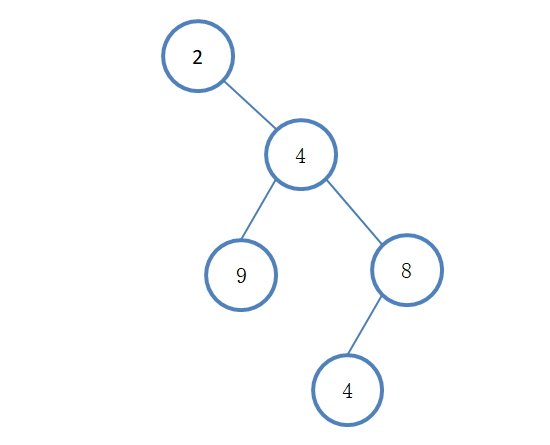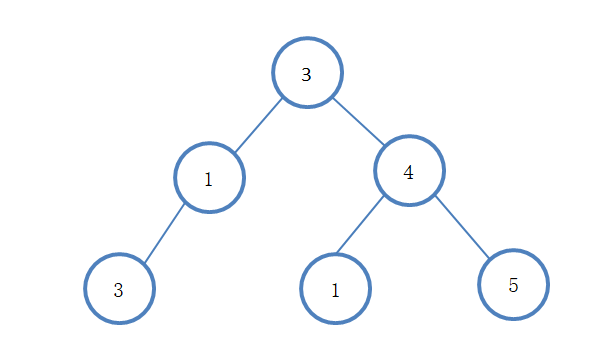### 树的结构

public class TreeNode {
public int val;
public TreeNode left;
public TreeNode right;
public TreeNode(int x) { val = x; }
}

### 实现代码

public class TreeNodeShow {

// 用于获得树的层数
private static int getTreeDepth(TreeNode root) {
return root == null ? 0 : (1 + Math.max(getTreeDepth(root.left), getTreeDepth(root.right)));
}

private static void writeArray(TreeNode currNode, int rowIndex, int columnIndex, String[][] res, int treeDepth) {
// 保证输入的树不为空
if (currNode == null) return;
// 先将当前节点保存到二维数组中
res[rowIndex][columnIndex] = String.valueOf(currNode.val);

// 计算当前位于树的第几层
int currLevel = ((rowIndex + 1) / 2);
// 若到了最后一层，则返回
if (currLevel == treeDepth) return;
// 计算当前行到下一行，每个元素之间的间隔（下一行的列索引与当前元素的列索引之间的间隔）
int gap = treeDepth - currLevel - 1;

// 对左儿子进行判断，若有左儿子，则记录相应的"/"与左儿子的值
if (currNode.left != null) {
res[rowIndex + 1][columnIndex - gap] = "/";
writeArray(currNode.left, rowIndex + 2, columnIndex - gap * 2, res, treeDepth);
}

// 对右儿子进行判断，若有右儿子，则记录相应的"\"与右儿子的值
if (currNode.right != null) {
res[rowIndex + 1][columnIndex + gap] = "\\";
writeArray(currNode.right, rowIndex + 2, columnIndex + gap * 2, res, treeDepth);
}
}

public static void show(TreeNode root) {
if (root == null){
System.out.println("EMPTY!");
return;
}
// 得到树的深度
int treeDepth = getTreeDepth(root);

// 最后一行的宽度为2的（n - 1）次方乘3，再加1
// 作为整个二维数组的宽度
int arrayHeight = treeDepth * 2 - 1;
int arrayWidth = (2 << (treeDepth - 2)) * 3 + 1;
// 用一个字符串数组来存储每个位置应显示的元素
String[][] res = new String[arrayHeight][arrayWidth];
// 对数组进行初始化，默认为一个空格
for (int i = 0; i < arrayHeight; i++) {
for (int j = 0; j < arrayWidth; j++) {
res[i][j] = " ";
}
}

// 从根节点开始，递归处理整个树
// res[(arrayWidth + 1)/ 2] = (char)(root.val + '0');
writeArray(root, 0, arrayWidth / 2, res, treeDepth);

// 此时，已经将所有需要显示的元素储存到了二维数组中，将其拼接并打印即可
for (String[] line : res) {
StringBuilder sb = new StringBuilder();
for (int i = 0; i < line.length; i++) {
sb.append(line[i]);
if (line[i].length() > 1 && i <= line.length - 1) {
i += line[i].length() > 4 ? 2 : line[i].length() - 1;
}
}
System.out.println(sb.toString());
}
}
}

### 测试用例

#### 测试一

TreeNode q = TreeNodeUtil.arrayToTreeNode(new Integer[]{2,null,4,9,8,null,null,4});
TreeNodeShow.show(q);

        2
\
4
/   \
9       8
/
4#### 测试二

TreeNode q = TreeNodeUtil.arrayToTreeNode(new Integer[]{3,1,4,3,null,1,5});
TreeNodeShow.show(q);

      3
/   \
1       4
/       / \
3       1   5